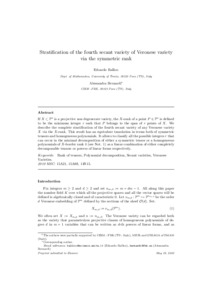# Stratification of the fourth secant variety of Veronese variety via the symmetric rank.

Ballico, Edoardo ; Bernardi, Alessandra (2010) Stratification of the fourth secant variety of Veronese variety via the symmetric rank. [Preprint]
Full text available as:Preview PDF Download (282kB) | Preview

## Abstract

If $X\subset \PP n$ is a projective non degenerate variety, the $X$-rank of a point $P\in \PP n$ is defined to be the minimum integer $r$ such that $P$ belongs to the span of $r$ points of $X$. We describe the complete stratification of the fourth secant variety of any Veronese variety $X$ via the $X$-rank. This result has an equivalent translation in terms both of symmetric tensors and homogeneous polynomials. It allows to classify all the possible integers $r$ that can occur in the minimal decomposition of either a symmetric tensor or a homogeneous polynomials of $X$-border rank $4$ (see Not. \ref{border}) as a linear combination of either completely decomposable tensors or powers of linear forms respectively.

Abstract
Document type
Preprint
Creators
CreatorsAffiliationORCID
Ballico, Edoardo
Bernardi, Alessandra
Keywords
Rank of tensors, Polynomial decomposition, Secant varieties, Veronese Varieties
Subjects
DOI
Deposit date
21 May 2010 08:03